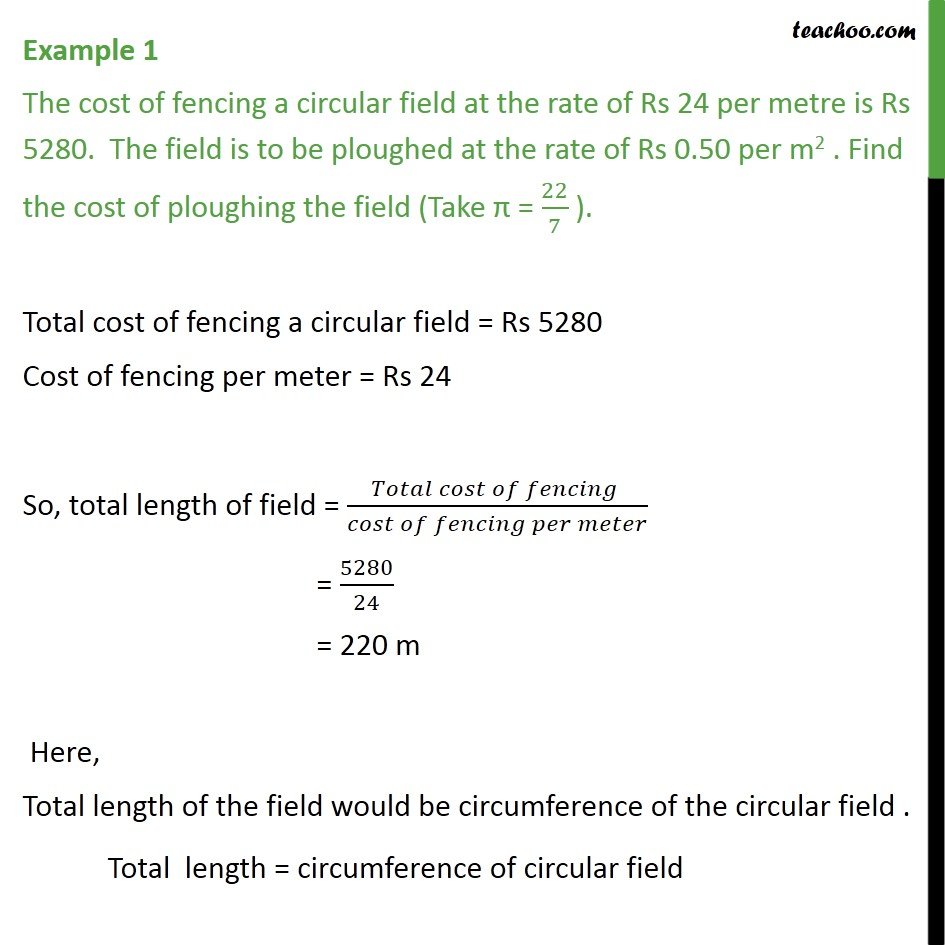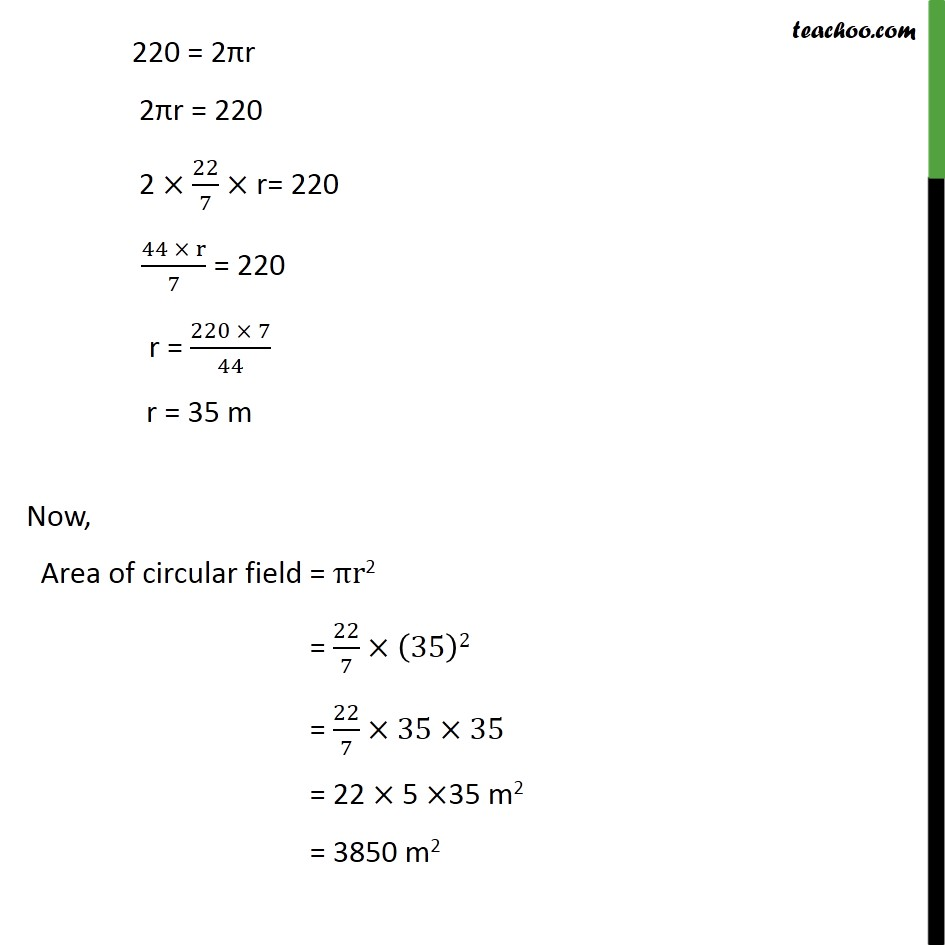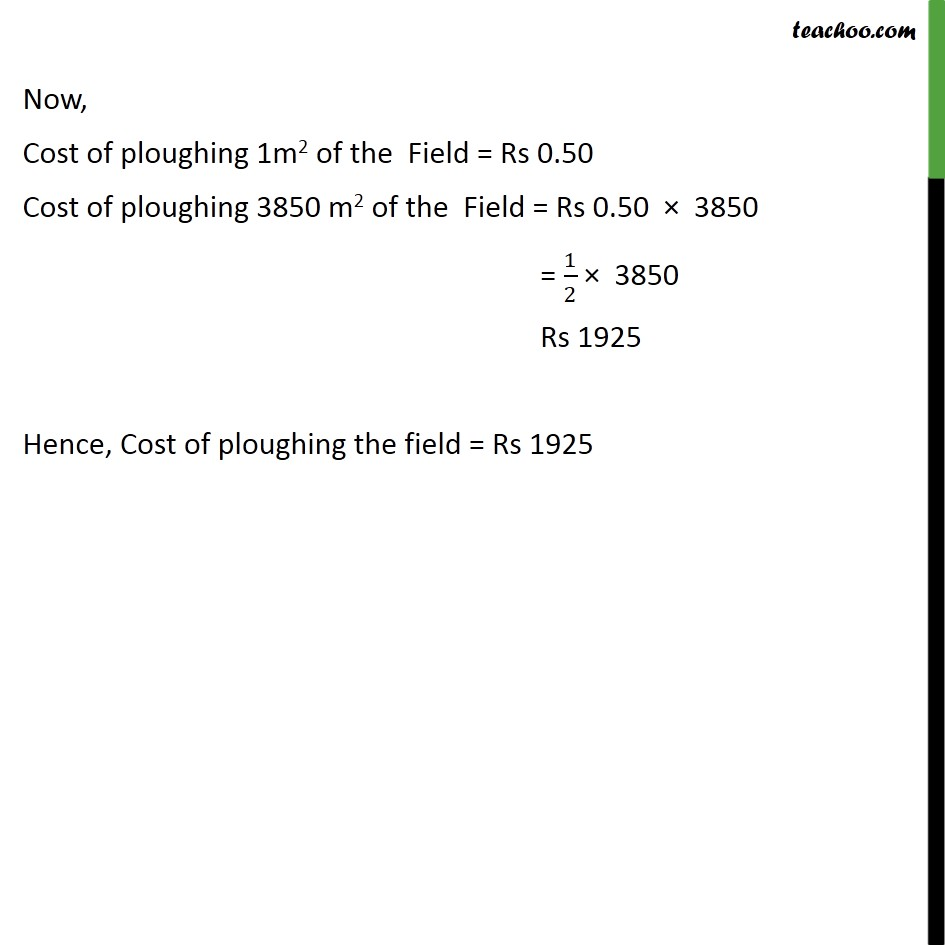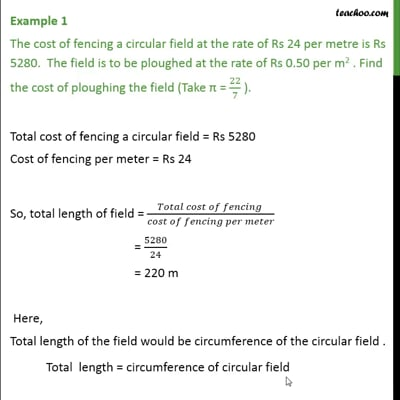Examples

Chapter 12 Class 10 Areas related to Circles
Serial order wiseThis video is only available for Teachoo black users

Solve all your doubts with Teachoo Black (new monthly pack available now!)

### Transcript

Example 1 The cost of fencing a circular field at the rate of Rs 24 per metre is Rs 5280. The field is to be ploughed at the rate of Rs 0.50 per m2 . Find the cost of ploughing the field (Take π = 22/7 ). Total cost of fencing a circular field = Rs 5280 Cost of fencing per meter = Rs 24 So, total length of field = (𝑇𝑜𝑡𝑎𝑙 𝑐𝑜𝑠𝑡 𝑜𝑓 𝑓𝑒𝑛𝑐𝑖𝑛𝑔 )/(𝑐𝑜𝑠𝑡 𝑜𝑓 𝑓𝑒𝑛𝑐𝑖𝑛𝑔 𝑝𝑒𝑟 𝑚𝑒𝑡𝑒𝑟) = 5280/24 = 220 m Here, Total length of the field would be circumference of the circular field . Total length = circumference of circular field 220 = 2πr 2πr = 220 2 ×22/7× r= 220 (44 × r)/7 = 220 r = (220 × 7)/44 r = 35 m Now, Area of circular field = πr2 = 22/7×(35)2 = 22/7×35×35 = 22 × 5 ×35 m2 = 3850 m2 Now, Cost of ploughing 1m2 of the Field = Rs 0.50 Cost of ploughing 3850 m2 of the Field = Rs 0.50 × 3850 = 1/2 × 3850 Rs 1925 Hence, Cost of ploughing the field = Rs 1925